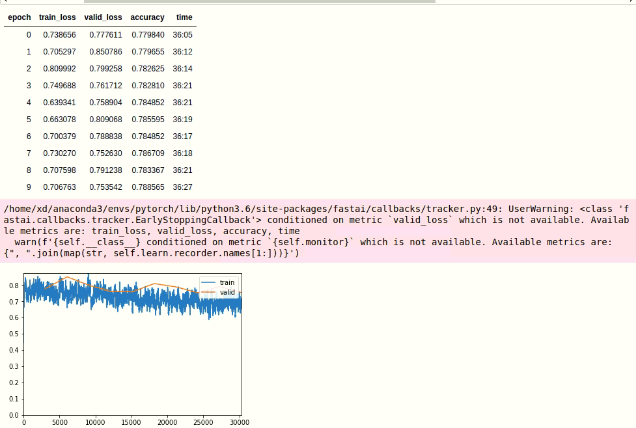# Confusing EarlyStoppingCallback docs

Hi, I was trying to use `EarlyStoppingCallback`

``````learn1 = cnn_learner(data1, get_senet154, cut=-3, split_on=lambda m: (m, m), metrics=[accuracy], callback_fns=[partial(EarlyStoppingCallback, monitor='accuracy', min_delta=0.01, patience=3)])
learn1.unfreeze()
rn50_stage2_callbacks = [CSVLogger(learn=learn1, filename=NAME+'stage2-history'),ShowGraph(learn=learn1)]
learn1.fit_one_cycle(5, slice(1e-5), callbacks=rn50_stage2_callbacks)
``````

as https://docs.fast.ai/callbacks.tracker.html#EarlyStoppingCallback says, `will automatically try to determine if the quantity should be the lowest possible (validation loss) or the highest possible (accuracy)`. but in my case it didn’t determine correctly.

I suppose the accuracy should be increasing? But the callback wants it to decrease… I wonder should I set mode to min or max? Thx in advance.

You should try with a lower delta. Here you always add 0.78… as accuracy and you want at least 0.78+0.01 to consider you have a better one. Try with 1e-4 instead?

1 Like

Oh i see, I mistakenly thought it had passed deltaThanks @sgugger I’ll change it to a lower delta!

@sgugger Will you please take a look at this…? I’ve got a more confusing situation …I wonder if I can use valid_loss as monitorThx!

1 Like

It’s hard to say without seeing the whole code you’re running and the full error message.

@sgugger My bad. I changed only accuracy to valid_loss for monitor.

``````train_il = ImageList.from_df(df=train_df, cols='Id', path=DATA_DIR/'train_images/')
# Category
src1 = (train_il.split_by_rand_pct(valid_pct=0.1, seed=233)
.label_from_df(cols='Category')
tfms = get_transforms(do_flip=True, max_zoom=1.1, max_rotate=12, xtra_tfms=[cutout(n_holes=(1,4), length=(20, 40), p=.75), jitter(magnitude=0.005, p=.25)])
data1 = (src1
.transform(tfms, size=200)
.databunch(path='.', bs=16, num_workers=8)
.normalize(imagenet_stats))
learn1 = cnn_learner(data1, get_senet154, cut=-3, split_on=lambda m: (m, m), metrics=[accuracy], callback_fns=[partial(EarlyStoppingCallback, monitor='valid_loss', min_delta=1e-3, patience=3)])Found the reason. Internally the metric is called `val_loss`, but it prints different names in the warning. Fixing so it’s `valid_loss`.